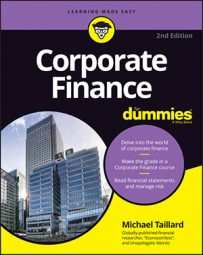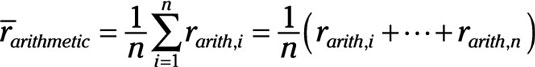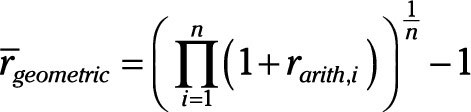##### Corporate Finance For DummiesThe degrees of success being generated by corporations can be measured in many ways. There are several different methods for evaluating the success of standard capital investments as well as financial or portfolio investments.

## Arithmetic rate of returns

The arithmetic rate of return on a specific asset is pretty simple. It’s a spot measurement that measures only the total rate of return over the life of the investment, like this:

R = (VtP)/P

where:

Vt = The value of the asset at time t
P = Purchase price

So, take the value of your asset at any point in history, subtract your purchase price to determine your gain or loss, and then divide that by your purchase price to determine the rate of return. It’s a very simple calculation that provides very important information about how well you’re utilizing your purchases, assets, investments . . . pretty much everything you own.

For those assets that don’t increase in value but produce things of value (such as machinery), you can include the value of those things produced as a part of your value at time, t.

A variation on this includes calculations that account for reinvestment of the cash flows generated on the investment, for those investments that generate cash flows or can be reinvested after maturity (for example, capital, bonds, dividend-generating stocks, and money market investments).

## Average rate of returns

The average rate of returns starts with the rate of return and measures that for every year you care about (the years you’re including in your calculation). You add up the rate of return from each year, then divide it by the number of years you’re measuring. That’s the average rate of return.

If you just happen to care, the calculation looks like this and makes the process much more intimidating than it actually is:## Time-weighted rate of returns

The average rate of return distributes all returns equally so that the rate is the same each year. Using the time-weighted approach gives you a better understanding about how performance changes by weighting the returns from each year being included. This calculation is done like so:This method of determining your rate of return is actually more accurate because it accounts for changes in the rate of return over time. It may look intimidating, but it really isn’t. Here’s how it’s done:

1. Find the rate of return from each year and add 1 to each.

3. Divide your answer from Step 2 by 1/n, where n is the number of years.

## Risk adjusted return on capital

The return on capital assets generated per dollar of economic capital is called the risk adjusted return on capital.

RAROC = Rate of Return/Economic Capital

Economic capital is the amount of liquid assets that a corporation must keep on hand to be able to handle risk concerns: credit risk, liquidity risk, and so on. So, this method of calculating the rate of return actually accounts for risk generated by measuring the amount or return per dollar of capital the corporation must keep on hand to compensate for the additional risk generated.